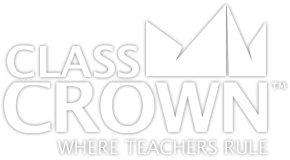# Geometry Worksheets

## Geometry Worksheets: Pack 1

Skills included in this pack:
• Identifying planar figures
• Identifying a shape/figure when described
• Identifying types of triangles as scalene, isosceles, or equilateral
• Identifying similar and congruent figures
• Identifying nets
• Identifying acute, obtuse, and right angles
• Identifying acute, obtuse, and right triangles
• Using a protractor to find the measure of an angle
• Estimating angle measures
• Finding missing angles using properties of adjacent angles
• Identifying lines of symmetry
• Identifying lines, line segments, and rays
• Identifying parallel, perpendicular, and intersecting lines
• Identifying rotations, reflections, and translations
• Finding unknown angles in triangles and quadrilaterals
• Identifying complementary and supplementary angles
• Identifying number of sides, vertices, edges, and faces of 3-dimensional figures
+ See More
Free Sample Pages Available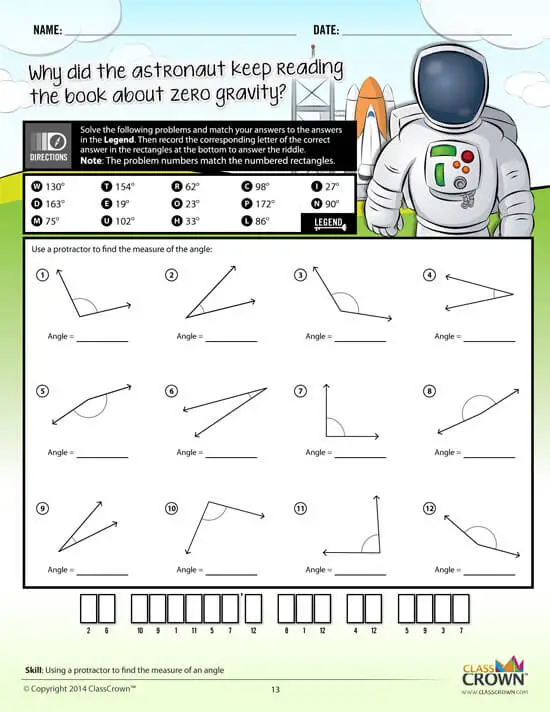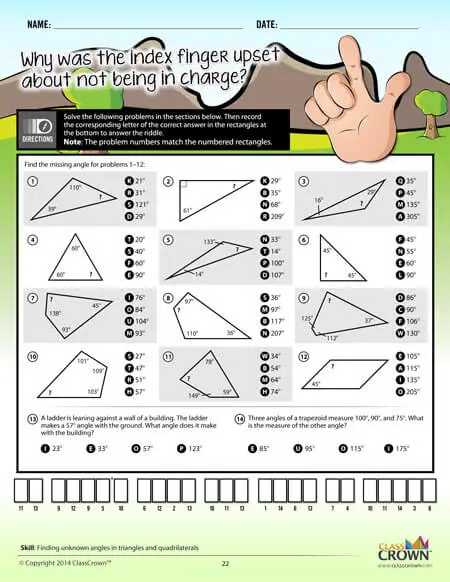## Geometry Worksheets: Pack 2

Skills included in this pack:
• Identifying parts of a circle
• Finding the perimeter of figures
• Finding the area of squares and rectangles
• Finding the area of triangles
• Finding the area of complex figures with right angles
• Finding the area between rectangles
• Finding the perimeter and area of rectangles, triangles, and complex figures with right angles
• Finding the volume of rectangular prisms
• Finding the radius and diameter of a circle
• Finding the area of a circle
• Finding the circumference and area of circles
• Finding the area of parallelograms and trapezoids
• Finding the circumference of a circle
• Finding surface area of cylinders, rectangular prisms, and right square pyramids
• Finding surface area with word problems
• Finding the volume and surface area of rectangular prisms
• Finding the volume and surface area of cylinders
+ See More
Free Sample Pages Available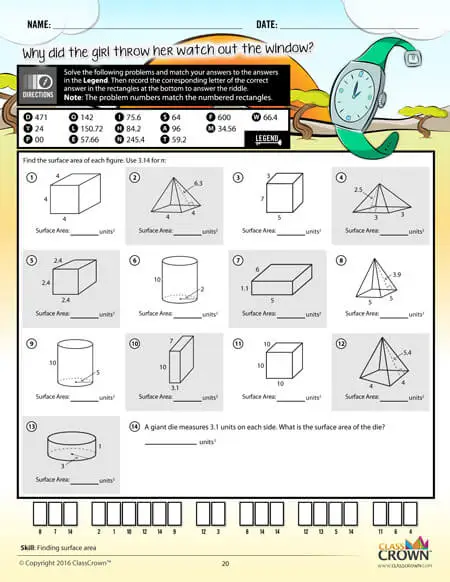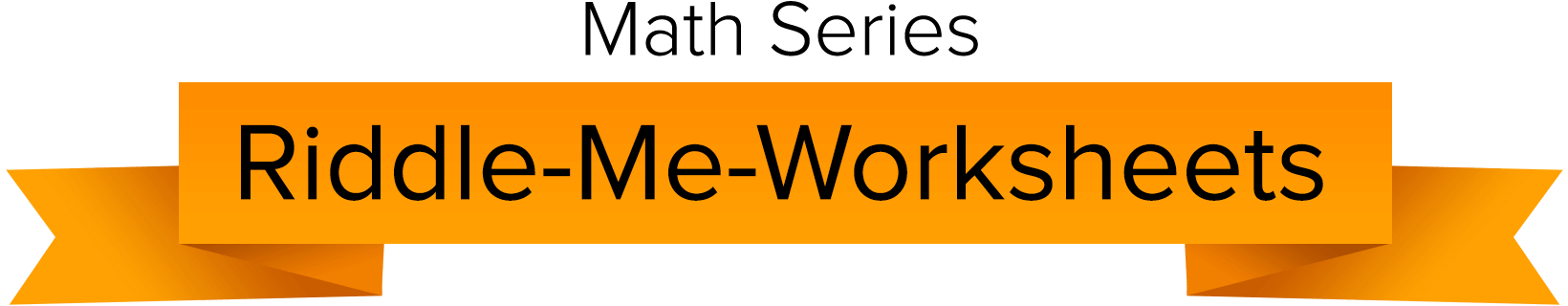Self Checking

Our math worksheets introduce a puzzle aspect to math, giving students immediate feedback as to whether or not they are solving problems correctly. If the answer to the riddle isn't spelled correctly, the student knows which problems he's made an error on.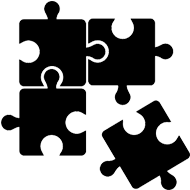Fun Puzzle AspectImmediate Feedback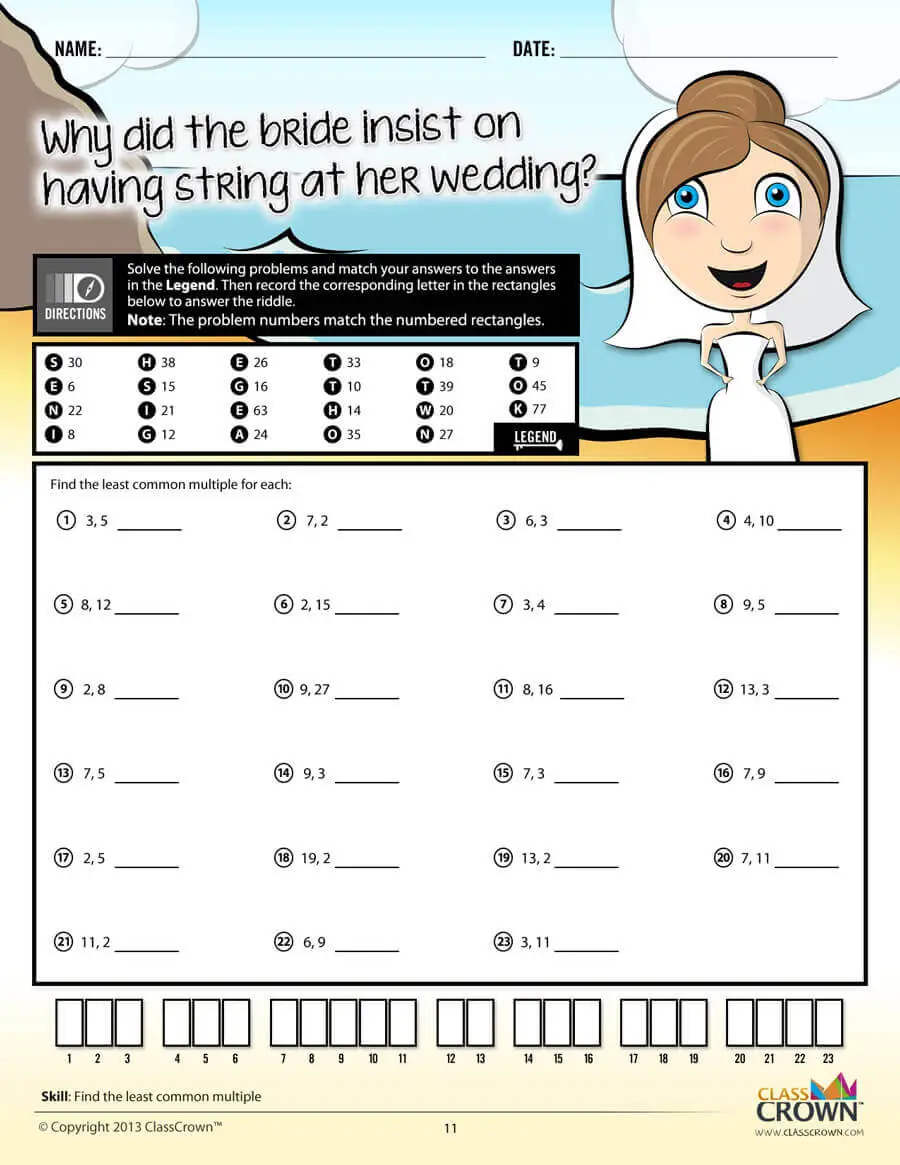Problem Solving Motivation

Each math worksheet contains a riddle that the student solves by completeing all the problems on the worksheet. This keeps kids motivated to complete each problem so that they can find the answer to the riddle.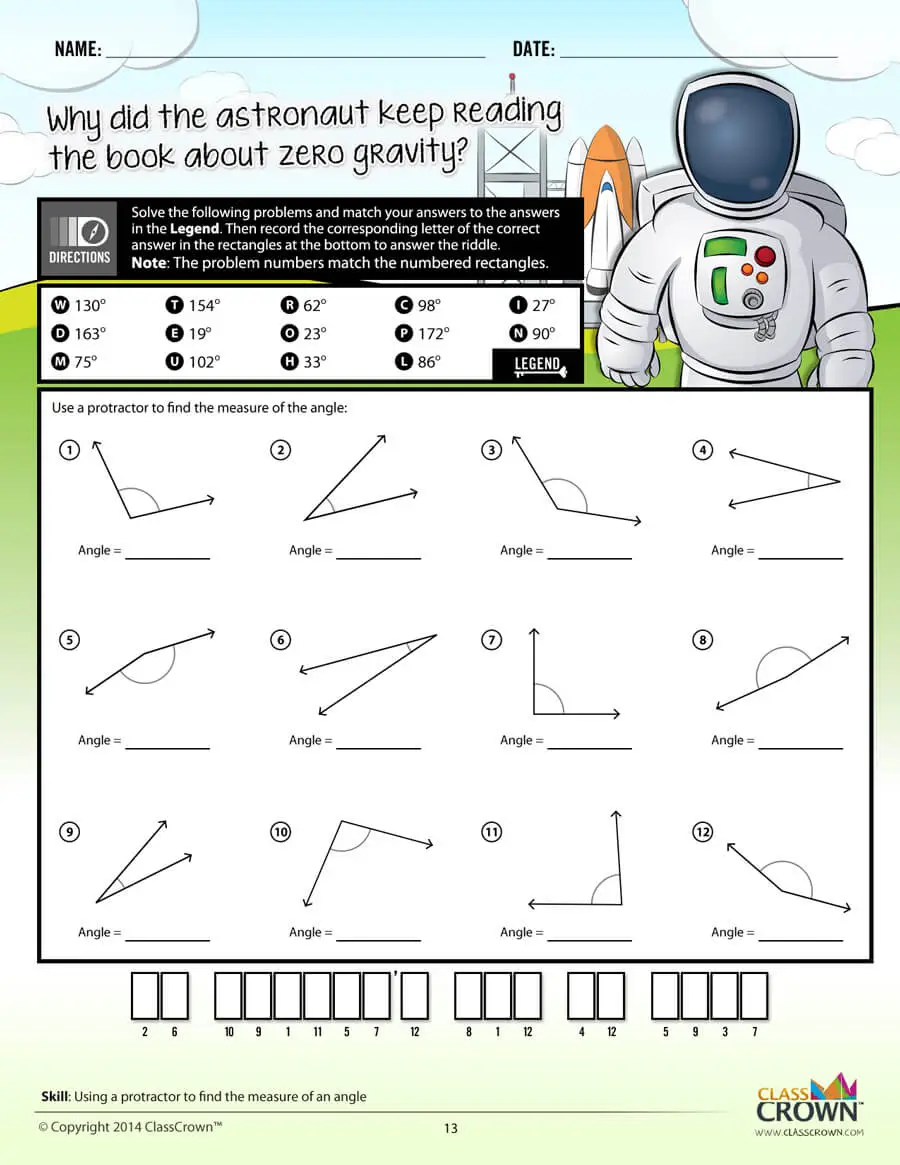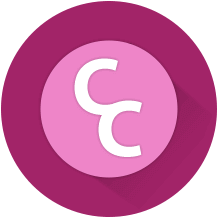Common Core Aligned

All our math worksheet packs are designed with Common Core in mind. That way you don’t have to worry about whether your math ciriculum is aligned or not when you incorpoate ClassCrown Riddle-Me-Worksheets in your lesson plans.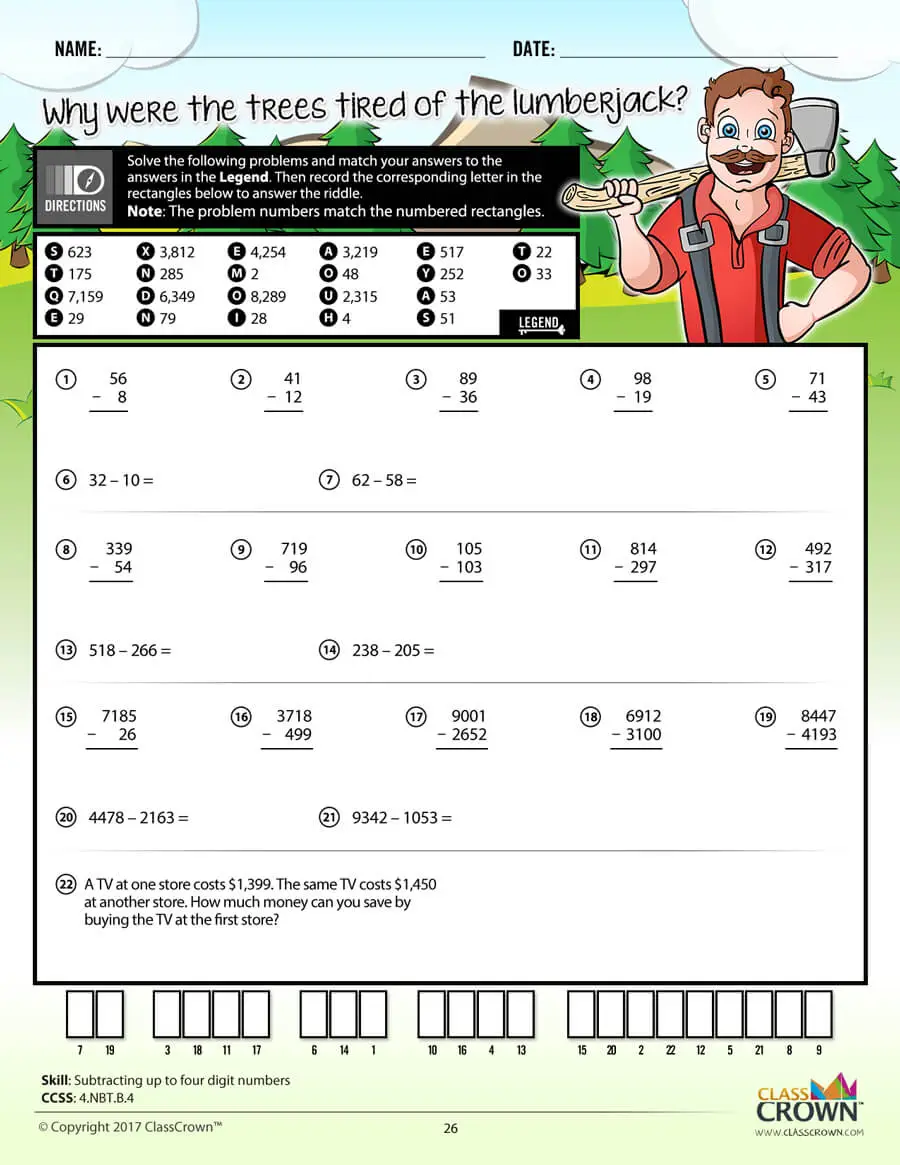High Quality Design

Each page of our math worksheets has been produced in high resolution at 144 dpi and designed in full, vibrant color for maximum quality. They look stunning whether you are printing in color or black and white.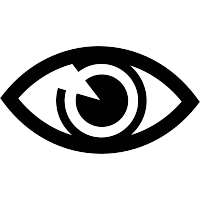High Resolution
(144 dpi)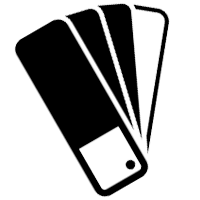Stunning Color & Clarity